# Krull's height theorem

## Statement

Let$R$ be a Noetherian commutative unital ring and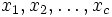$x_1, x_2, \ldots, x_c$ be elements in$R$. Let$P$ be minimal among primes containing all the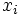$x_i$s. Then, the codimension of$P$ is at most$c$.

There is also a converse of this statement viz converse of Krull's height theorem.

## Proof

### Starting assumptions

Replacing$R$ by$R_P$ if necessary, we may assume that$R$ is a local ring with unique maximal ideal$P$.

In particular, we see that the ring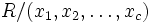$R/(x_1,x_2,\ldots,x_c)$ is a local Artinian ring with unique maximal ideal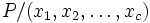$P/(x_1,x_2,\ldots,x_c)$, hence$P$ is nilpotent modulo$(x_1,x_2,\ldots,x_c)$.

### Main proof

Supose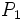$P_1$ is a prime contained in$P$, with no primes between. Then, it suffices to show, inductively, that$P_1$ is minimal over an ideal generated by$c-1$ elements.